# Maths - Axis-Angle to Quaternion - Sample Orientations

## Sample Rotations

In order to try to explain things and give some examples we can try I thought it might help to show the rotations for a finite subset of the rotation group. We will use the set of rotations of a cube onto itself, this is a permutation group which gives 24 possible rotations as explaned on this page.

In the following table we will need to know what quadrant the results are in, so I have taken some sample results from Math.atan2

heading applied first giving 4 possible orientations:reference orientation angle = 0 degrees axis = 1,0,0 qx = ax * sin(angle/2) qy = ay * sin(angle/2) qz = az * sin(angle/2) qw = cos(angle/2) q = 1rotate by 90 degrees about y axis angle = 90 degrees axis = 0,1,0 qx = ax * sin(angle/2) qy = ay * sin(angle/2) qz = az * sin(angle/2) qw = cos(angle/2) q = 0.7071 + j 0.7071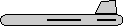rotate by 180 degrees about y axis angle = 180 degrees axis = 0,1,0 qx = ax * sin(angle/2) qy = ay * sin(angle/2) qz = az * sin(angle/2) qw = cos(angle/2) q = jrotate by 270 degrees about y axis angle = 90 degrees axis = 0,-1,0 or angle = -90 degrees axis = 0,1,0 qx = ax * sin(angle/2) qy = ay * sin(angle/2) qz = az * sin(angle/2) qw = cos(angle/2) q = 0.7071 - j 0.7071 (equivilant rotation to: -0.7071 + j 0.7071)

Then apply attitude +90 degrees for each of the above: (note: that if we went on to apply bank to these it would just rotate between these values, the straight up and streight down orientations are known as singularities because they can be fully defined without using the bank value)angle = 90 degrees axis = 0,0,1 qx = ax * sin(angle/2) qy = ay * sin(angle/2) qz = az * sin(angle/2) qw = cos(angle/2) q = 0.7071 + k 0.7071angle = 120 degrees axis = 0.5774,0.5774,0.5774 qx = ax * sin(angle/2) qy = ay * sin(angle/2) qz = az * sin(angle/2) qw = cos(angle/2) q = 0.5 + i 0.5 + j 0.5 + k 0.5angle = 180 degrees axis = 0.7071,0.7071,0 qx = ax * sin(angle/2) qy = ay * sin(angle/2) qz = az * sin(angle/2) qw = cos(angle/2) q = i 0.7071 +j 0.7071angle = 120 degrees axis = -0.5774,-0.5774,0.5774 qx = ax * sin(angle/2) qy = ay * sin(angle/2) qz = az * sin(angle/2) qw = cos(angle/2) q = 0.5 - i 0.5 - j 0.5 + k 0.5

Or instead apply attitude -90 degrees (also a singularity):angle = 90 degrees axis = 0,0,-1 (equivilant rotation to: angle = -90 degrees axis = 0,0,1) qx = ax * sin(angle/2) qy = ay * sin(angle/2) qz = az * sin(angle/2) qw = cos(angle/2) q = 0.7071 - k 0.7071 (equivilant rotation to: -0.7071 + k 0.7071)angle = 120 degrees axis = -0.5774,0.5774,-0.5774 qx = ax * sin(angle/2) qy = ay * sin(angle/2) qz = az * sin(angle/2) qw = cos(angle/2) q = 0.5 - i 0.5 + j 0.5 - k 0.5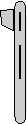angle = 180 degrees axis = -0.7071,0.7071,0 qx = ax * sin(angle/2) qy = ay * sin(angle/2) qz = az * sin(angle/2) qw = cos(angle/2) q = -i 0.7071 + j 0.7071 (equivilant rotation to: i 0.7071 - j 0.7071)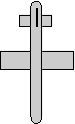angle = 120 degrees axis = 0.5774,-0.5774,-0.5774 qx = ax * sin(angle/2) qy = ay * sin(angle/2) qz = az * sin(angle/2) qw = cos(angle/2) q = 0.5 + i 0.5 - j 0.5 - k 0.5

Normally we dont go beond attitude + or - 90 degrees because thes are singularities, instead apply bank +90 degrees:angle = 90 degrees axis = 1,0,0 qx = ax * sin(angle/2) qy = ay * sin(angle/2) qz = az * sin(angle/2) qw = cos(angle/2) q = 0.7071 + i 0.7071angle = 120 degrees axis = 0.5774,0.5774,-0.5774 qx = ax * sin(angle/2) qy = ay * sin(angle/2) qz = az * sin(angle/2) qw = cos(angle/2) q = 0.5 + i 0.5 + j 0.5 - k 0.5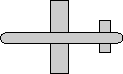angle = 180 degrees axis = 0,0.7071,-0.7071 qx = ax * sin(angle/2) qy = ay * sin(angle/2) qz = az * sin(angle/2) qw = cos(angle/2) q = j 0.7071 - k 0.7071angle = 120 degrees axis = 0.5774,-0.5774,0.5774 qx = ax * sin(angle/2) qy = ay * sin(angle/2) qz = az * sin(angle/2) qw = cos(angle/2) q = 0.5 + i 0.5 - j 0.5 + k 0.5

Apply bank +180 degrees:angle = 180 degrees axis = 1,0,0 qx = ax * sin(angle/2) qy = ay * sin(angle/2) qz = az * sin(angle/2) qw = cos(angle/2) q = iangle = 180 degrees axis = 0.7071,0,-0.7071 qx = ax * sin(angle/2) qy = ay * sin(angle/2) qz = az * sin(angle/2) qw = cos(angle/2) q = i 0.7071 - k 0.7071angle = 180 degrees axis = 0,0,1 qx = ax * sin(angle/2) qy = ay * sin(angle/2) qz = az * sin(angle/2) qw = cos(angle/2) q = kangle = 180 degrees axis = 0.7071,0,0.7071 qx = ax * sin(angle/2) qy = ay * sin(angle/2) qz = az * sin(angle/2) qw = cos(angle/2) q = i 0.7071 + k 0.7071

Apply bank -90 degrees:angle = 90 degrees axis = -1,0,0 (equivilant rotation to: angle = -90 degrees axis = 1,0,0) qx = ax * sin(angle/2) qy = ay * sin(angle/2) qz = az * sin(angle/2) qw = cos(angle/2) q = 0.7071 - i 0.7071 (equivilant rotation to: -0.7071 + i 0.7071)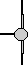angle = 120 degrees axis = -0.5774,0.5774,0.5774 qx = ax * sin(angle/2) qy = ay * sin(angle/2) qz = az * sin(angle/2) qw = cos(angle/2) q = 0.5 - i 0.5 + j 0.5 + k 0.5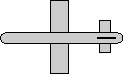angle = 180 degrees axis = 0,0.7071,0.7071 qx = ax * sin(angle/2) qy = ay * sin(angle/2) qz = az * sin(angle/2) qw = cos(angle/2) q = j 0.7071 + k 0.7071angle = 120 degrees axis = -0.5774,-0.5774,-0.5774 qx = ax * sin(angle/2) qy = ay * sin(angle/2) qz = az * sin(angle/2) qw = cos(angle/2) q = 0.5 - i 0.5 - j 0.5 - k 0.5